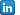January 2019

by @mw

Faster estimation of fixed-effect probit

Popularity of binary choice models makes their applications more and more sophisticated, reaching beyond the simple low-dimension applications. The implications of having plenty of independent variables may be severe, making the likelihood function highly irregular or even impossible to be solved. Even if the local maximum can be found, it is likely to be unstable or it may take a lot of time and computing power, especially if the independent variables are simple dummies.

Stata
`probit`
function tries to address some of the above these issues. For instance, the algorithm will check which observations do not bring extra information to the model and leave them out from the estimation. However, this step can be taking too long for some of the Big Data problems. A quick solution is to tell Stata in advance which observations to exclude when running the probit. Here is a simple plug and play code snippet.

Firstly, I define a generic setup with one dependent variable
`Yvar`
, some independent variables given by
`Xvars`
, and a set of fixed effects spanned by variable
`strata`
.
```/*
general setup
the variables must be specified in global macros as
Yvar   - dependent variable (binary)
Xvars  - independent vars
strata - factor variable (like country-sector-year)
*/
global Yvar   VARNAME
global Xvars  VARNAME(S)
global strata VARNAME```
Secondly, I run the standard
`probit`
estimation and allow Stata to find and treat fixed effects. I will later compare the timing of that procedure to the one proposed below.
```*standard estimation
timer on 1
xi: probit \\$Yvar \\$Xvar i.\\$strata
timer off 1```
The basic strategy is to exclude the observations which are not used in the estimation before running the
`probit`
command. It includes overall three steps, which adjust the sample selection variable
`modelSelect`
. It will be equal to 0 if the observations are to be excluded and 1 otherwise.

Firstly, remove the observations which have either missing
`Yvar`
or
`Xvars`
. Secondly, exclude the stratas for which there is no variability in the dependent variable. For this I check if the standard deviation of
`Yvar`
is 0. Lastly, create a vector of adjusted fixed effects for there is non-zero variability in
`Yvar`
. The number of adjusted fixed effects is smaller or equal to their original number. This is where the efficiency gains come from.
```*efficient estimation
timer on 2

*missing Yvar
gen modelSelect = !missing(\\$Yvar)

*missing Xvar
foreach var of global \\$Xvars {
replace modelSelect = 0 if missing(`var`)
}

*variability
bys \\$strata: egen sd_y = sd(\\$Yvar) if modelSelect == 1
replace modelSelect = 0 if sd_y == 0
drop sd_y

*exclude redundant factors
levelsof \\$strata if modelSelect==1, local(slevs)
egen match = anymatch(\\$strata), values(1 `slevs`)
gen sub_\\$strata = match * \\$strata
replace sub_\\$strata = . if sub_\\$strata == 0
drop match
xi I.sub_\\$strata, prefix(_E2)

probit \\$Yvar \\$Xvars _E* if modelSelect == 1
timer off 2```
As the last step I compare the execution times of both methods.
```timer list 1 2
```

As a quick demonstration I take a sample of European firms making investment decision, which is a dependent dummy variable. There is a total of ten independent variables and some 400 strata variables, comprising 407k observations. The overall file size was around 1gb, including variables not used in the model. (Unfortunately, I cannot disclose the data points.)

This simple trick reduced the computational time from 465.88 to 58.58 seconds, so by a factor of 8. Clearly, the efficiency gains are substantial and they increase with the data size and complexity of the setup.Marcin Wolski, PhD
Economist
European Investment Bank
E-mail: M.Wolski (at) eib.org
Phone: +352 43 79 88708View my profileIDEAS/RePEc

Categories